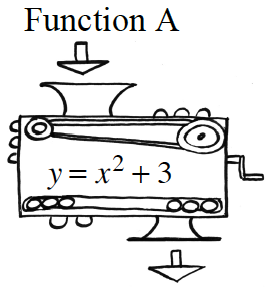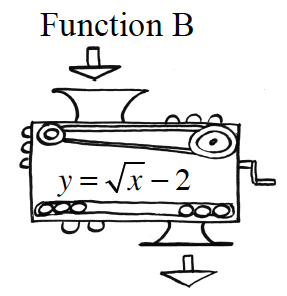### Home > CCA > Chapter 1 > Lesson 1.1.2 > Problem1-22

1-22.

Function Machines

1. If an input of $-9$ is put into each of the machines at right, what is each output?

Substitute $-9$ for $x$ in each of the equations in both the machines, then solve the equations for $y$.

2. Eric wants to get an output of $0$. Can he do this with each machine? If so, how? If not, why not?

What numbers could Eric substitute in the equations to get $0$?
Is it possible to get this answer from both machines?

He cannot get an output of 0 with Relation A.  He can get an output of 0 by putting a 4 in Function B